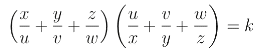Let P = u:v:w be a point and k a real number. The generalized Tucker cubic T(P, k) is the cubic with equation :T(P, k) is a non-pivotal cubic nK(#P, P) with pole the barycentric square Ω of P and root P. Its equation may be written under the following equivalent forms : (1) : ∑ v w x^2 (w y + v z) + (3 - k) u v w x y z= 0 or ∑ u^2 y z (w y + v z) + (3 - k) u v w x y z = 0, (2) : ∑ u x (w y - v z)^2 + (9 - k) u v w x y z = 0. (3) : ∑ u x (w y + v z)^2 - (k + 3) u v w x y z = 0, *** Singular cases and consequences • T(P, 0) decomposes into the trilinear polar L(P) of P and the circum-conic C(P) with perspector P. • T(P, 1) decomposes into the sidelines of the anticevian triangle of P. It follows that any cubic T(P, k) is a member of the pencil of circum-cubics generated by these two decomposed cubics. For a given P, all these cubics pass through the traces U, V, W of L(P) on the sidelines of ABC and the tangents at A, B, C to T(P, k) also contain U, V, W. If Q is another point, the isoconjugation that swaps P and Q maps T(P, k) onto T(Q, k). The pencil of cubics generated by T(P, k) and T(Q, k) contains the pivotal cubic with pole Ω and pivot the intersection of L(P) and L(Q).Special cases • T(P, k) contains P if and only if k = 9 and then, T(P, 9) is cK0(#P, P), a nodal cubic with node P. This is obvious from equation (2). For instance, K015 = T(X2, 9), K228 = T(X1, 9), K229 = T(X6, 9). • T(P, 3) is a nK0, see equation (1). For instance, K016 = T(X2, 3). • T(P, 2) is also remarkable. For instance, K327 = T(X2, 2).Circular cubics T(P, k) can be a circular cubic (for some k) if and only if P lies on the Grebe cubic K102 = pK(X6, X6). Two isogonal conjugates on K102 correspond to two cubics, each being the isogonal transform of the other. In particular, when P = X(1), both cubics coincide giving the isogonal focal cubic K721.Equilateral cubics T(P, k) can be an equilateral cubic (for some k) if and only if P lies on K278 = pK(X1989, X1989). The corresponding cubics are very complicated.Other remarkable cubics See the related cubics K214 and K721.